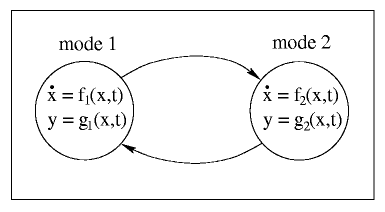MSc Thesis Proposal

# Optimal control of linear hybrid systems with saturation

Mentors: B. De Schutter

Prerequisites: research oriented attitude of the student

Keywords: hybrid systems, control, optimization

Description:
Hybrid systems arise from the interaction between continuous variable systems (i.e., systems that can be described by a difference or differential equation) and discrete event systems (i.e., systems where the state transitions are initiated by events that occur at discrete time instants). In general we could say that a hybrid system can be in one of several "regimes" whereby in each regime the behavior of the system can be described by a system of difference or differential equations, and that the system switches from one regime to another due to the occurrence of events (see Figure 1).Figure 1: A schematic representation of a hybrid system with two regimes.

Up to now there are no useful modeling and control techniques that can be applied to general hybrid systems and that are still tractable. Therefore, we consider a special class of hybrid systems in this research proposal: the class of linear hybrid systems with saturation. In each regime the evolution of such a system can be described by linear difference or differential equations, possibly with saturation at some upper or lower bounds. Typical examples of this kind of systems are traffic light controlled intersections or systems consisting of several fluid containers that are connected by tubes with valves.
The control objective is to determine the optimal switching time instants from one regime to another. In some cases this problem can be solved analytically whereas in other cases numerical optimization is required to determine the optimal switching scheme. The aim of this research proposal is to develop efficient methods to compute optimal switching schemes for (subclasses of) linear hybrid systems with saturation.

If you are interested in selecting this project as your MSc project, please come along or send us an email for more information.